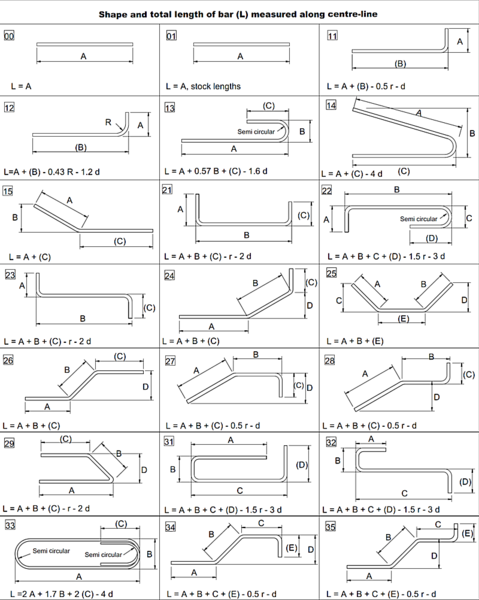### Bar Bending schedule (BBS)

The bar bending schedule is a table that contains the details of reinforcement for a specific structural member such as footing, column, slab, and others. The bar bending schedule will contain the bars' dimensions to be used, the shape of the bars, the size of bars, bar mark, number of bars, and the weight of bars. BBS will be used to determine the weight of reinforcement required for a specific structural member.

there are various advantages of bar bending schedule such as:

1. estimating the quantity of reinforcement for each structural member. So we can estimate the amount of reinforcement required for a construction project.
2. BBS will help in reducing the losses of steel by procuring the precise quantity of different rebar diameter.
3. BBS will reduce the wastage of reinforcement by providing the engineers with the exact dimension and shape of rebars. Knowing the exact dimension and shape will reduce the time of cutting and bending rebars.
4. BBS will reduce the effort at the time of billing. Quantities of reinforcement will be calculated and shown in BBS. Therefore the billing of reinforcement for various members will be quick and simple.
5. For big projects. The main contractor will not cut and bend the reinforcement. The cutting and bending will be done at the factory. The presence of BBS will reduce the effort and time of cutting and bending reinforcement at the factory.
6. The bar marks can help the fabricators in placing the rebars in correct locations. The bar mark usually is shown in the shop drawing, and each bundle of bars will have a tag with a bar mark and detail.
The preparation of the bar bending schedule will be done for different bars in a reinforced member. First, we will identify an identical rebar group. From figure no:1, we can see a different type of rebar—for example, T32-125-B1. T23 is the bar diameter, and it will place at a space of 125 mm. B1 is the location of the bar. The legend of reinforcement drawing will explain the symbols such as B1, B2, T1, etc. after determining the identical group of bars such as T32-125-B1, we will give the group a unique id such as 1A1.

The next step is to determine the number of bars in each group. The number of bars can be determined by knowing the spacing between bars, length of the member, and the concrete cover. For our example: we have T32-125-B1. The C-C spacing between 32 bars is 125 mm, and the length is 9000 mm, as shown clearly in figure no:2. The concrete cover is 100 mm.

number of bars=(9000-2*100)/125+1=71.4 so it is 72 bars.
So now we have the mark for this group of bars(1A1), and we have the number of bars(72).

After determining the bar mark and bar number, we need to determine the bar length. Bar length will depend on bar shape.usually, the designer will determine the bar shape. If we look at figure no:1 again. We will notice that the bar T25-150-B2 is a U shape as drawn by the designer. Similarly, T32-125-B1is a U shape, but it is not shown clearly in this section. Usually, the designer will provide a plan and a cross-section in each direction. We can also notice that the designer determined the shape of bar T32-250-FF, which is a dowel bar for the wall above the pile cap. The shape of this bar is L, the length of each shape can be determined from figure no:3. While calculating the bar's length, we should not forget the overlap length with other bars. for long members, it is impossible to use an individual bar from the start to end. Therefore, we use two or more bars, and we overlap it. Overlapping will be determined by the designer. The designer will usually provide the overlapping length and anchorage length, similar to what is shown in figure no:4.Figure 3

Figure 4

The last step is to calculate the weight of each group of bars. the weight will be calculated using the following equation:
weight of bar group=(no of bars)*(length of the bar)*(Bar weight per meter)
bar weight per meter for various bar diameters shown in figure no:4. Figure no:5 showing a sample of the bar bending schedule.